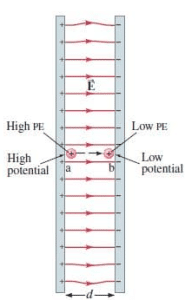Mechanics

# Difference Between Electric Potential and Electric Potential Energy

The basic difference between electric potential and electric potential energy is that Electric potential at a point in an electric field is the amount of work done to bring the unit positive charge from infinity to that point, while electric potential energy is the energy that is needed to move a charge against the electric field.

The gravitational potential at a point in the gravitational field is the gravitational potential energy of a unit mass placed at that point. In this way, the electric potential at any point in the electric field is the electric potential energy of a unit positive charge at that point.

If W is the work done in moving a unit positive charge q from infinity to a certain point in the field, the electric potential V at this point is given by:

V = W/q
It implies that electric potential is measured relative to some reference point and like potential energy we can measure only the change in potential between two points.

Electric potential is the scalar quantity. Its unit is volt which is equal to joule per coulomb (J/C).

### Definition of volt

If one joule of work is done against the electric field to bring the unit positive charge from infinity to the point in the electric field then the potential difference at that point will be one volt.

## Electric potential energy

To apply the law of conservation of energy, we need to define electric potential energy, potential energy can be defined only for conservative force. The work done by a conservative force in moving an object between any two positions is independent of the path taken. The electrostatic force between any two charges is conservative because the dependence of on positions is just like the gravitational force which is a conservative force. Hence we can define potential energy for electrostatic force.
We know that the change in potential energy between any two points, a, and b equals the negative of work done  by the  conservative force on an object as it moves from point a to point b:

Δ P.E = -W

Hence we define the change in potential energy (P.Eb – P.E a), when a point charge q moves from some point a to another point b.As the negative of the work done by the electric force on charge as it moves from point a to b.
For Example, consider the electric field between two equally but oppositely charged parallel plates, we assume their separation is small compared to their width and height, so the field will be uniform over most of the region as shown in the figure:Now consider the tiny positive charge q placed at point “a” very near to the positive plate. This charge q is so small that it has no effect on electric field E. If this charge q at point a is released, the electric force will work on the charge and accelerate it towards the negative plate. Work done by the electric field E to move the charge at a distance d is:

W= Fd =-qed

In this case, the electric field is uniform. In the case which is illustrated above potential difference decreases, as the charged particle moves from point a to b, and the particle’s kinetic energy increases by an equal amount.

According to the law of conservation of energy, electric potential energy is transformed into kinetic energy, and total energy is conserved. Note that the positive charge q has its greatest potential energy at point a, near the positive plate. The reverse is true for a negative charge, its potential energy is greatest near the negative plate.

Video about the difference between electric potential and electric potential energy

Related Topics:
See related Topics on physics in the links given below:

### Related Articles

1. PUNEET RATHOUR says:

as per your definitions, the electric potential is the amount of work done to move a unit positive test charge from infinity to any point under the electric field. and electric potential energy is the amount of energy needed to move test charge against the electric field. okay, no problem yet. now come to your example:- the electric field is acting force on small charge and this force takes that charge to a negative plate. it means here work is done by the electric field. okay, the electric potential is defining here. but what about electric potential energy here? what is the source of energy here for a small charge? and it is not going even against the electric field? the electric field applies force on it and then kinetic energy comes to it so that it can move to another point. but what about electric potential energy? I am not seeing it anywhere here?

1. Ken Bone says:

smol pp

2. Concerned Professor says:

This is very wrong: “The basic difference between electric potential and electric potential energy is that Electric potential at a point in an electric field is the amount of work done to bring the unit positive charge from infinity to that point, while electric potential energy is the energy that is needed to move a charge against the electric field.” My students are finding this and getting confused. It would be great if it were taken down, or fixed. See any basic physics text, like the ones from openstax, for example.